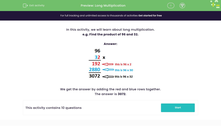# Understand Long Multiplication

In this worksheet, students find the product of two two-digit numbers.Key stage:  KS 2

Curriculum topic:   Number: Multiplication and Division

Curriculum subtopic:   Multiply Numbers to Four Digits

Difficulty level:#### Worksheet Overview

In this activity, we will learn about long multiplication.

First of all, let's recap on how to do simple multiplication

How would we find out the answer to 96 x 2?

We would start by doing 6 x 2 = 12

We put the 2 in the answer box in the ones column and carry the 1 forwards to the tens column.

Then we do 9 x 2 = 18.  Don't forget to add the 1 that was carried from the ones column and we have 19.

Write 19 in the answer box and we have the full answer of 192

So 96 x 2 = 192

Now let's test ourselves further and try out some long multiplication:

e.g. Find the product of 96 and 32

Remember that find the product simply means multiply.We get the answer by adding the red and blue rows together.